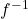## What is the inverse of the function f(x) = one-quarterx – 12?

Question

What is the inverse of the function f(x) = one-quarterx – 12?

in progress 0
1 month 2021-08-13T20:18:10+00:00 2 Answers 0 views 0(x) = 4x + 48

Step-by-step explanation:

let y = f(x) and rearrange making x the subject, that is

y =x – 12 ( multiply through bt 4 to clear the fraction )

4y = x – 48 ( add 48 to both sides )

4y + 48 = x

Change t back into terms of x with x =(x)(x) = 4x + 48